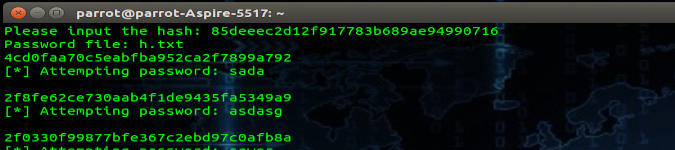# The Linux Rain Linux General/Gaming News, Reviews and Tutorials# How to Bruteforce a Hash from Windows Hash File in Python

#### By Kalyani Rajalingham, published 23/05/2020 in Tutorials

Passwords are stored as hashes and as such even if a hacker breaks into and steals a database, every password will be in the form of a hash, which is not reversible. Hashes may not be reversed, but they definitely can be brute forced! Hashes can be brute forced using packages on GitHub like the rainbow tables, but building a brute forcer in Python is just as simple.

First let's import the two modules required from Python.

``````import hashlib
import sys``````

We then collect two sets of data from the user:

(I) The hash to crack

``````inp = input("Please input the hash: ")
passfile = input("Password file: ") ``````

Next, we need a function that will hash the passwords in the password file. This function's purpose is to take an input of some kind and chuck out its hash value. In this example, we use md5 as the hash type, however, we can use just about anything including NTLM.

For MD5:

``````def hasher(inputt):
hashh = hashlib.md5()
hashh.update(inputt.encode())
ret = hashh.hexdigest()
return ret ``````

For NTLM:

``````def hasher(inputt):
hashh = hashlib.new('md4', inputt.encode('utf-16le'))
ret = hashh.hexdigest()
return ret``````

Now that we have our hashing function, the rest is easy. We just need to open up the file with the passwords, and for each password, we apply the hashing function and turn it into a hash, and compare the hash value with the value of the hash we retrieved or wish to crack.

``````fil = open(passfile, 'r')

for word in fil:

k =word.strip('\n')
bb= hasher(k)
if bb == inp:
print('[*] Hash found: %s ' % (word))
break
else:
print('[*] Attempting password: %s ' % (word))``````

The code as a whole would look something like this:

``````import hashlib
import sys

inp = str(input("Please input the hash: "))

def hasher(inputt):

hashh = hashlib.new('md4', inputt.encode('utf-16le')).hexdigest()
return hashh

fil = open(passfile, 'r')

for word in fil:

k =word.strip('\n')
bb= hasher(k)
if bb == inp:
print('[*] Hash found: %s ' % (word))
break
else:
print('[*] Attempting password: %s ' % (word))``````

Happy Hacking!

[Editor's Note/Disclaimer: Information in the above article was for education and ethical hacking practices]